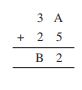# Q1. Find the values of the letters in each of the following and give reasons for the steps involved.Here we are adding two numbers and unit place of the first number and the second number is A and  5 respectively. unit place of the answer is 2 so the way we can get this result is when we get 12 on adding unit places of both number i.e.

A + 5 = 12

which implies      A = 12 - 5 = 7.

Ten's digit of both numbers are 3 and 2.remainder=1

so ten's digit of the answer(B) = 3 + 2 + 1 = 6

Hence A= 7 and B = 6.

## Related Chapters

### Preparation Products

##### JEE Main Rank Booster 2021

This course will help student to be better prepared and study in the right direction for JEE Main..

₹ 13999/- ₹ 9999/-
##### Rank Booster NEET 2021

This course will help student to be better prepared and study in the right direction for NEET..

₹ 13999/- ₹ 9999/-
##### Knockout JEE Main April 2021 (Easy Installments)

An exhaustive E-learning program for the complete preparation of JEE Main..

₹ 4999/-
##### Knockout NEET May 2021

An exhaustive E-learning program for the complete preparation of NEET..

₹ 22999/- ₹ 14999/-